VisualVM is a great tool for exploring Java applications and the JVM in which they are running. One area which has always been a bit overwhelming (to me) is the heap dump - a mass of raw information for millions of objects, which can be hard (for me) to sift through.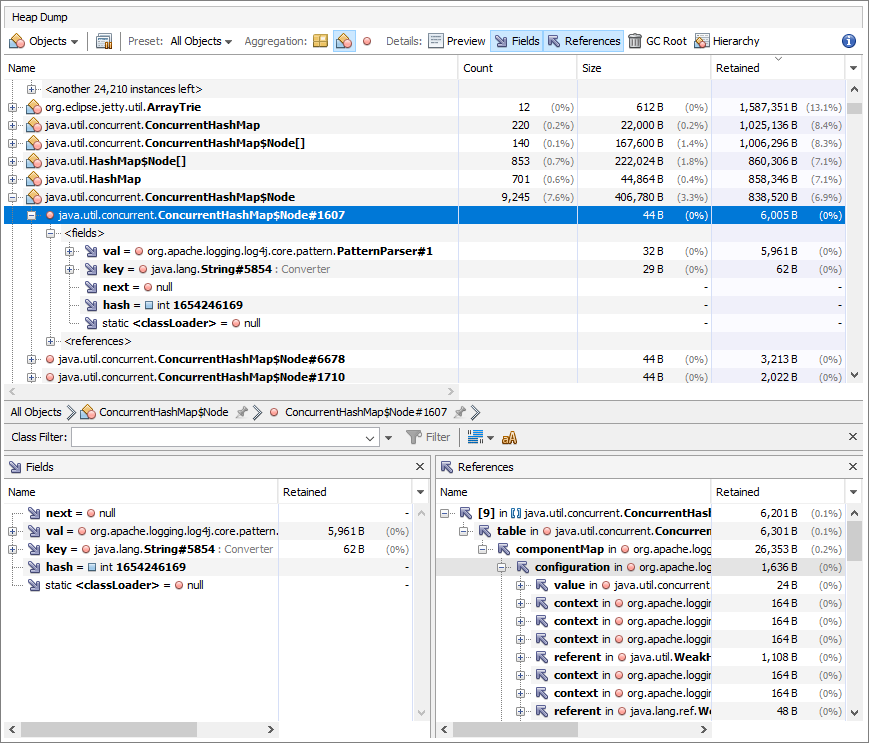OQL (Object Query Language), a tool which is included in the heap analyzer, allows you to filter heap data in a variety of ways.

As the help pages explain, OQL is based on JavaScript expression language, and includes a set of built-in objects and functions. An expression is a JavaScript fragment which produces a value.

By combining these objects and functions with JavaScript expressions, you can write and execute complex queries against your heap data. The help page (referenced above) contains lots of examples - but I will show some specific ones below.

Here is a simple example to get us started: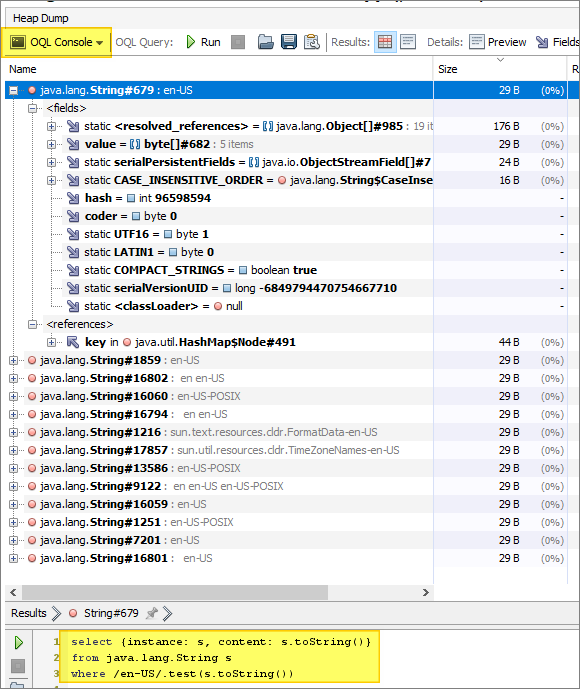And here is the query from the above example:

 ``````1 2 3 `````` ``````select {instance: s, content: s.toString()} from java.lang.String s where /en-US/.test(s.toString()) ``````

The above query uses two expressions.  The select clause contains an expression which creates a JavaScript object literal.  The object defines two properties - instance and content:

``````{instance: s, content: s.toString()}
``````

The above expression refers to a variable (s) which is defined in the from clause:

``````java.lang.String s
``````

Finally, the where clause consists of a JavaScript test() expression - which returns true or false. This is our filter - just like a SQL where clause.

The end result is a query which returns all String objects containing “en-US”. (This example uses a regular expression, so the text we’re searching for can be a substring.)

OQL queries can be plain JavaScript expressions - you don’t have to use the OQL “select…” syntax: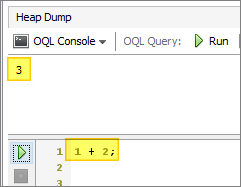The semicolon at the end of the expression is optional.

Here is a more interesting example: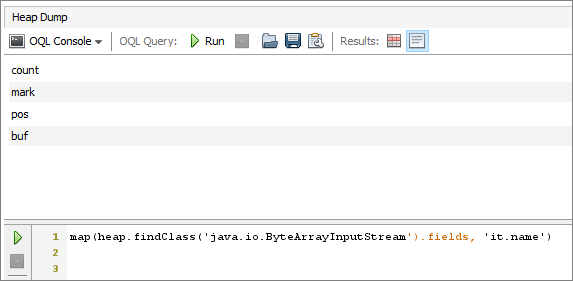The above example could also have been written using the select keyword (a select statement which does not use a from clause or a where clause):

``````select map(heap.findClass('java.io.ByteArrayInputStream').fields, 'it.name')
``````

What does the above query do, and how does it work?

It uses the OQL map() function, which takes two parameters:

1. an array (or iterable)
2. an expression string (or a callback function)

The array is created by using the fields property of another built-in OQL function - the `heap.findClass()` function.  This returns an array of (non-static) fields for the specified class.

The expression string is indeed a string representing a JavaScript expression. In the above example, the expression refers to “it” which is a built-in variable of the `map()` function, representing the current element in the array over which `map()` iterates.

Each element in our array is a field object - and each OQL field object has two properties: `name` and `signature`. In our example, we access the name property using the expression string `it.name`.

The end result is a list of the four fields (count, mark, pos, and buf) of the `ByteArrayInputStream` class.

Taking this one step further, I mentioned above that `map()` takes an expression string or a callback function. Here is that same example again, but this time using a callback instead of an expression string:

 ``````1 2 3 4 5 `````` ``````map(heap.findClass('java.io.ByteArrayInputStream').fields, function (it) { var res = ''; res += toHtml(it.name) + " : " + toHtml(it.signature); return res + "
"; }); ``````

In the above example, we access both properties of the field object - name and signature. We also use the OQL toHtml() function to build our results.

The output looks like this: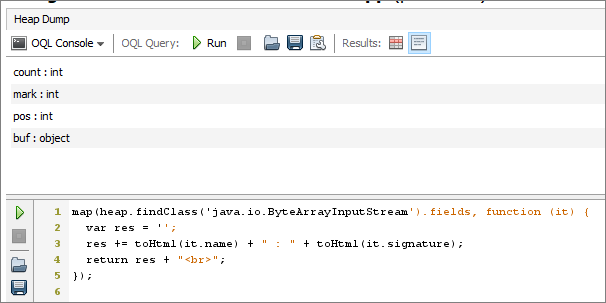One more example:

 ``````1 2 3 `````` ``````select map(sort(heap.objects('java.net.URI'), 'lhs.string.toString().localeCompare(rhs.string.toString())'), 'toHtml(it) + " : " + it.string.toString()') ``````

This sorts a list of URI objects by their string values.

There are several more advanced examples of OQL statements in the help page - and even more on the web (Stack Overflow is a good source).